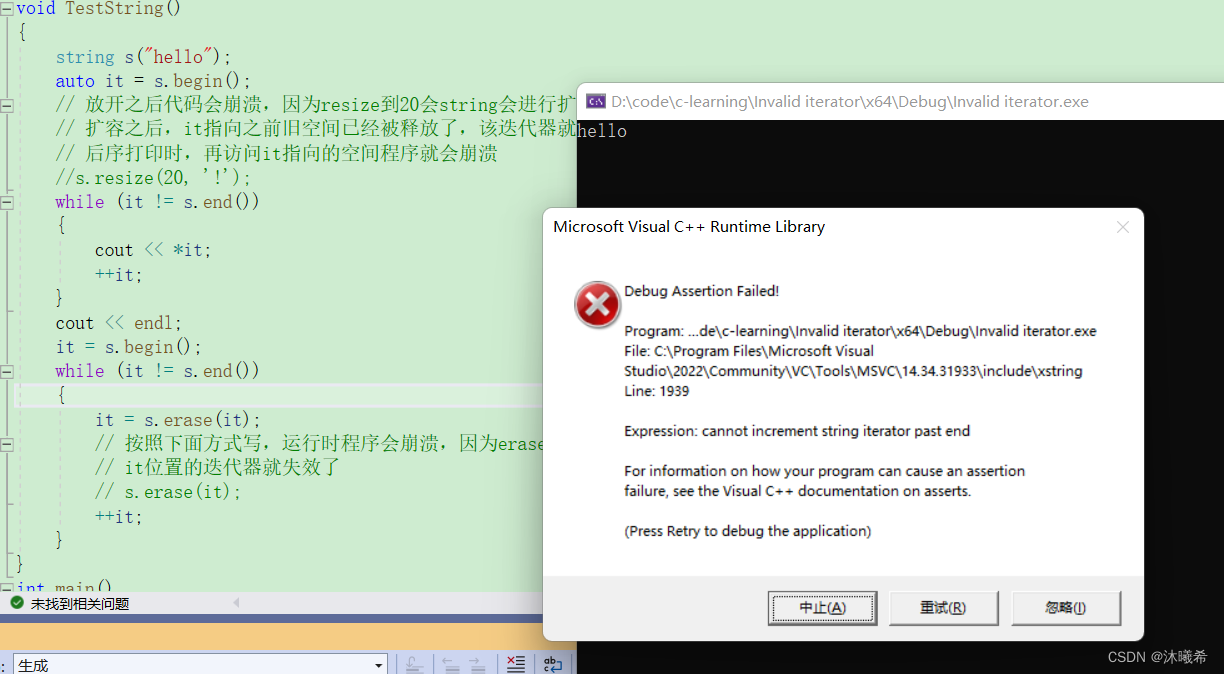dd

deeplab

# 一.vector介绍

1. vector是表示可变大小数组的序列容器。
2. 就像数组一样，vector也采用的连续存储空间来存储元素。也就是意味着可以采用下标对vector的元素进行访问，和数组一样高效。但是又不像数组，它的大小是可以动态改变的，而且它的大小会被容器自动处理。
3. 本质讲，vector使用动态分配数组来存储它的元素。当新元素插入时候，这个数组需要被重新分配大小为了增加存储空间。其做法是，分配一个新的数组，然后将全部元素移到这个数组。就时间而言，这是一个相对代价高的任务，因为每当一个新的元素加入到容器的时候，vector并不会每次都重新分配大小。
4. vector分配空间策略：vector会分配一些额外的空间以适应可能的增长，因为存储空间比实际需要的存储空间更大。不同的库采用不同的策略权衡空间的使用和重新分配。但是无论如何，重新分配都应该是对数增长的间隔大小，以至于在末尾插入一个元素的时候是在常数时间的复杂度完成的。
5. 因此，vector占用了更多的存储空间，为了获得管理存储空间的能力，并且以一种有效的方式动态增长。
6. 与其它动态序列容器相比（deque, list and forward_list）， vector在访问元素的时候更加高效，在末尾添加和删除元素相对高效。对于其它不在末尾的删除和插入操作，效率更低。比起list和forward_list统一的迭代器和引用更好。

# 二、构造函数

(constructor)构造函数声明 接口说明
vector()（重点） 无参构造
vector（size_type n, const value_type& val = value_type()） 构造并初始化n个val
vector (const vector& x); （重点） 拷贝构造
vector (InputIterator first, InputIterator last); 使用迭代器进行初始化构造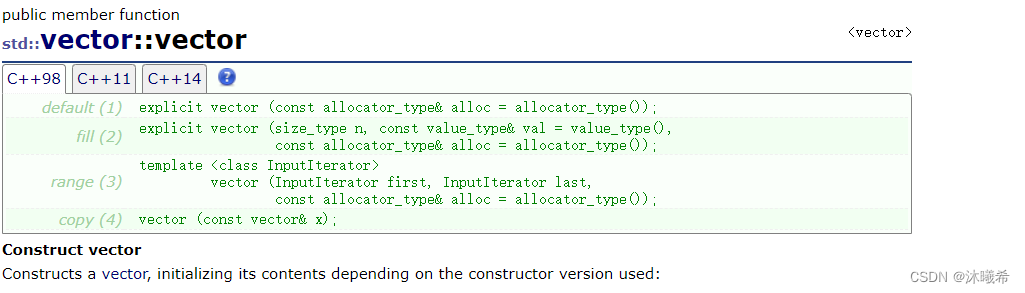``````void test_vector1()
{
vector<int> v1;  //无参构造
vector<int> v2(5, 1);  //构造并初始化5个1
vector<int> v3(v2);  //拷贝构造
vector<int> v4(v3.begin(), v3.end());  //使用迭代器进行初始化构造
}
``````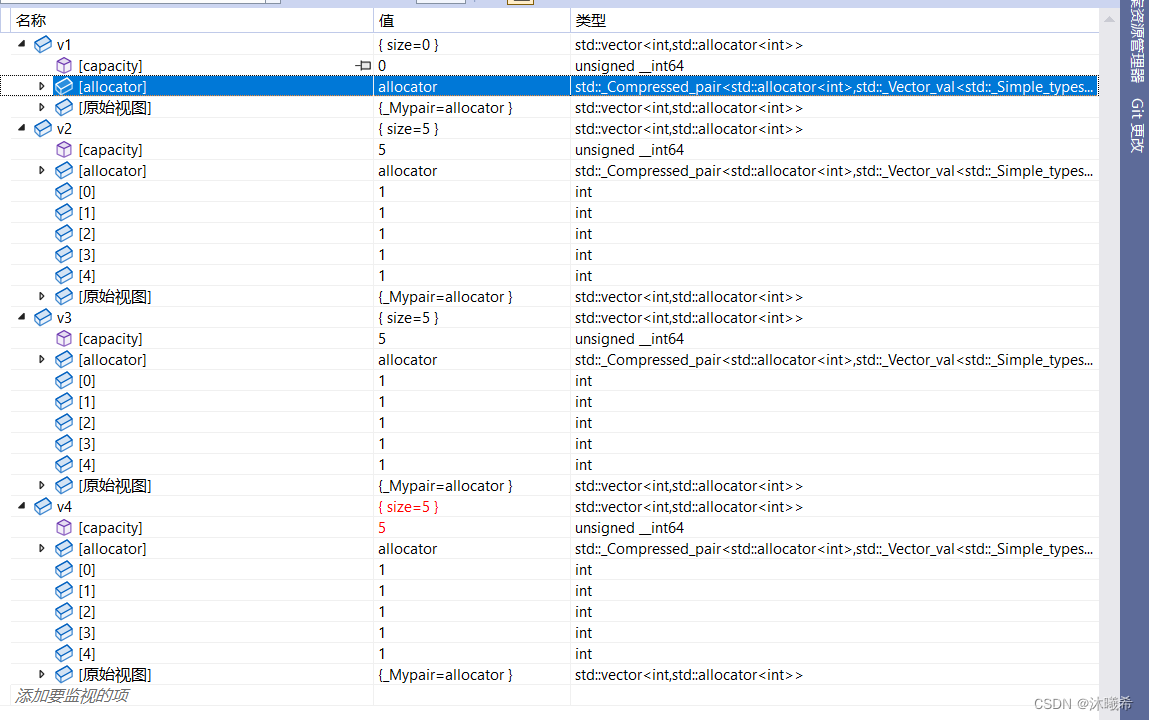# 三、遍历

## 1.[]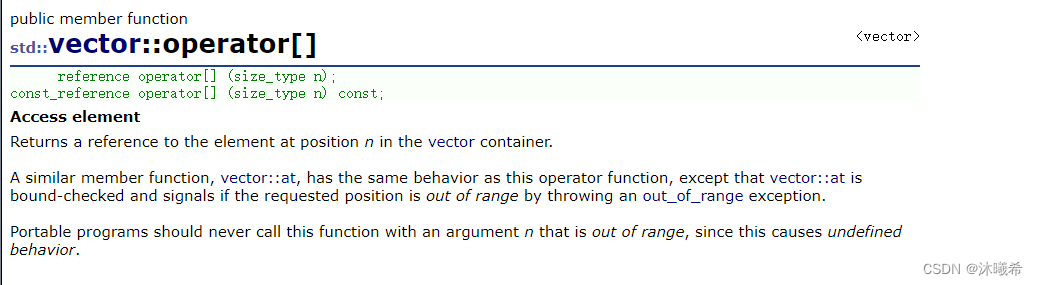JavaSE

``````void test(const vector<int>& v)
{
for (size_t i = 0; i < v.size(); ++i)
{
//只读
cout << v[i] << " ";
}
cout << endl;
}
void test_vector2()
{
vector<int> v1;
v1.push_back(1);
v1.push_back(2);
v1.push_back(3);
v1.push_back(4);
v1.push_back(5);
for (size_t i = 0; i < v1.size(); ++i)
{
//可读可写
++v1[i];
cout << v1[i] << " ";
}
cout << endl;
test(v1);
}
``````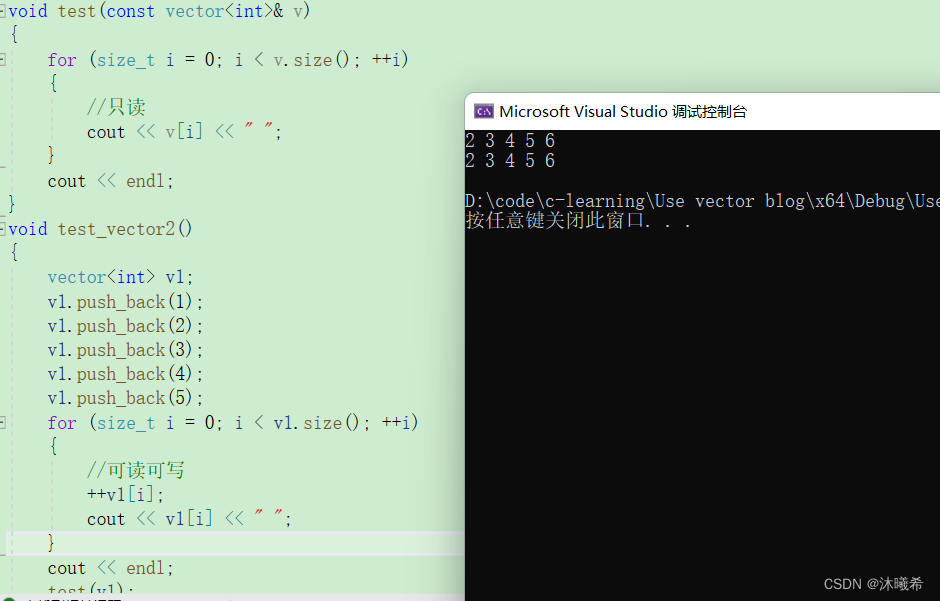## 2.迭代器

iterator的使用 接口说明
begin +end（重点） 获取第一个数据位置的iterator/const_iterator， 获取最后一个数据的下一个位置的iterator/const_iterator
rbegin + rend 获取最后一个数据位置的reverse_iterator，获取第一个数据前一个位置的reverse_iterator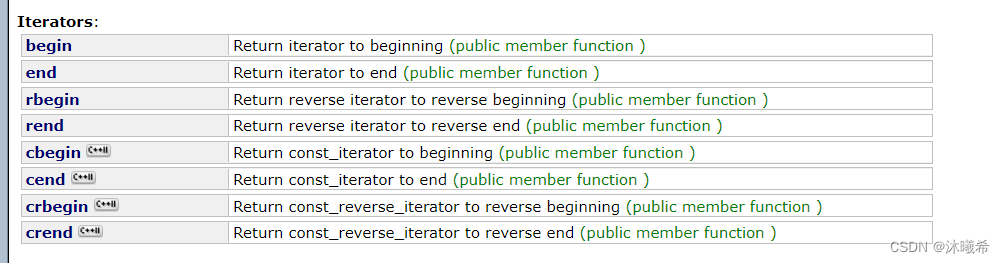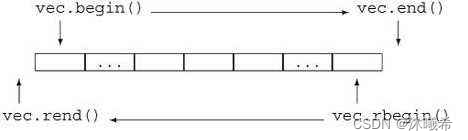``````void PrintVector(const vector<int>& v)
{
// const对象使用const迭代器进行遍历打印
vector<int>::const_iterator it = v.begin();
while (it != v.end())
{
cout << *it << " ";
++it;
}
cout << endl;
}

void test_vector3()
{
// 使用push_back插入4个数据
vector<int> v;
v.push_back(1);
v.push_back(2);
v.push_back(3);
v.push_back(4);

// 使用迭代器进行遍历打印
vector<int>::iterator it = v.begin();
while (it != v.end())
{
cout << *it << " ";
++it;
}
cout << endl;

// 使用迭代器进行修改
it = v.begin();
while (it != v.end())
{
*it *= 2;
++it;
}

// 使用反向迭代器进行遍历再打印
// vector<int>::reverse_iterator rit = v.rbegin();
auto rit = v.rbegin();
while (rit != v.rend())
{
cout << *rit << " ";
++rit;
}
cout << endl;

PrintVector(v);
}
``````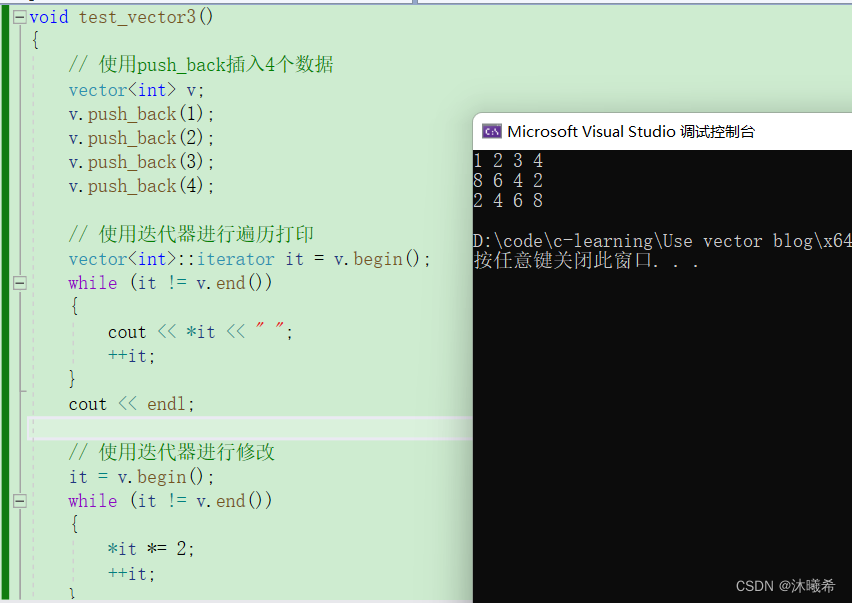awk 命令使用

## 3.范围for

``````void test1(const vector<int>& v)
{
for (auto e : v)
{
//只读
cout << e << " ";
}
cout << endl;
}
void test_vector4()
{
vector<int> v;
v.push_back(1);
v.push_back(2);
v.push_back(3);
v.push_back(4);
for (auto e : v)
{
//可读可写
++e;
cout << e << " ";
}
cout << endl;
test1(v);
}
``````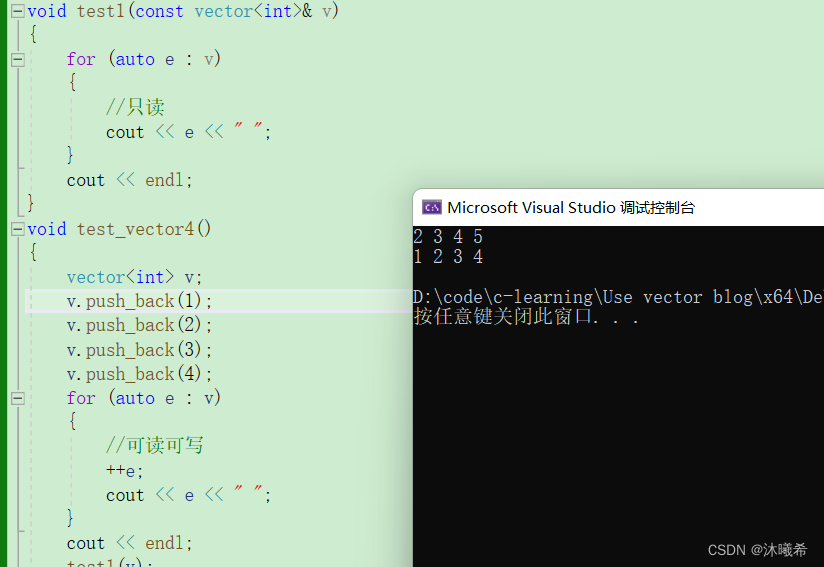# 四、容量操作

size 获取数据个数
capacity 获取容量大小
empty 判断是否为空
resize（重点） 改变vector的size
reserve （重点） 改变vector的capacity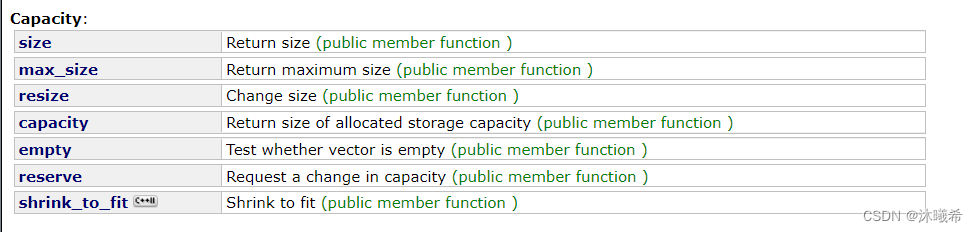gson

``````void test_vector5()
{
vector<int> v;
v.push_back(1);
v.push_back(2);
v.push_back(3);
v.push_back(4);
v.push_back(5);
for (auto e : v)
{
cout << e << " ";
}
cout << endl;
cout << v.size() << endl;
cout << v.capacity() << endl;
v.resize(10, 1);
for (auto e : v)
{
cout << e << " ";
}
cout << endl;
cout << v.size() << endl;
cout << v.capacity() << endl;
v.resize(3, 1);
for (auto e : v)
{
cout << e << " ";
}
cout << endl;
cout << v.size() << endl;
cout << v.capacity() << endl;
v.reserve(10);
for (auto e : v)
{
cout << e << " ";
}
cout << endl;
cout << v.size() << endl;
cout << v.capacity() << endl;
v.reserve(3);
cout << v.size() << endl;
cout << v.capacity() << endl;
}
``````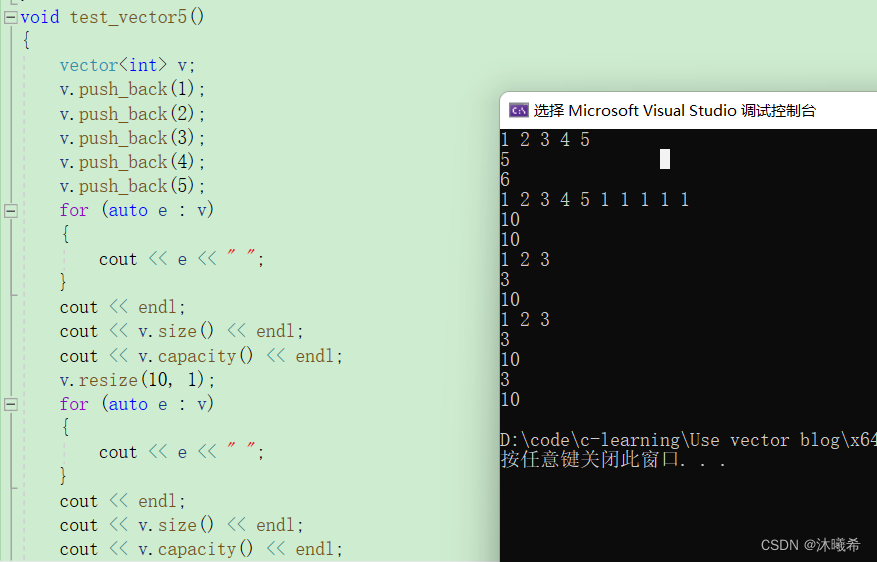## 1.扩容机制

capacity的代码在vs和g++下分别运行会发现，vs下capacity是按1.5倍增长的，g++是按2倍增长的。具体增长多少是根据具体的需求定义的。vs是PJ版本STL，g++是SGI版本STL。

``````void test_vector6()
{
size_t sz;
vector<int> v;
sz = v.capacity();
cout << "making v grow:\n";
for (int i = 0; i < 100; ++i)
{
v.push_back(i);
if (sz != v.capacity())
{
sz = v.capacity();
cout << "capacity changed: " << sz << '\n';
}
}
}
``````

vs下: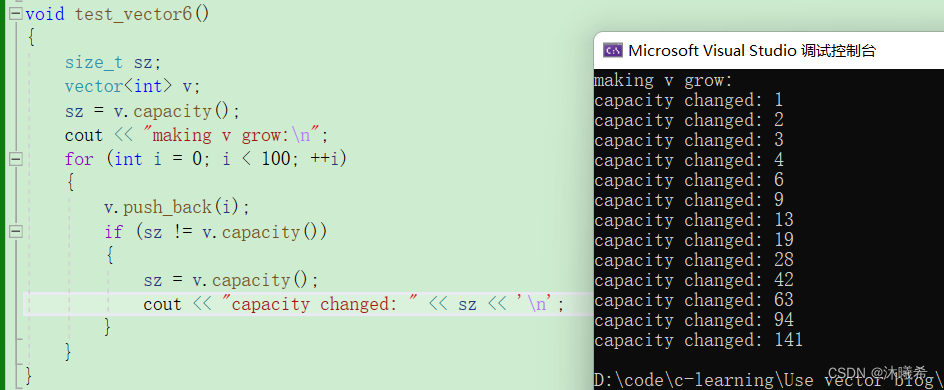Linux的g++下: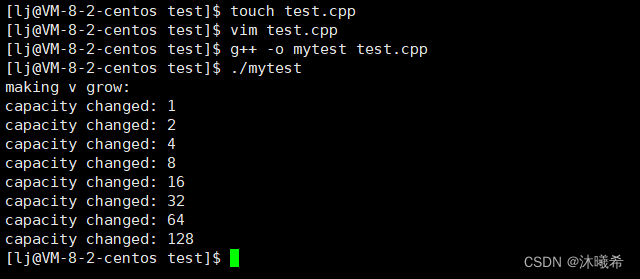Swift开发

# 五、增删查改

vector增删查改 接口说明
push_back（重点） 尾插
pop_back （重点） 尾删
find 查找。（注意这个是算法模块实现，不是vector的成员接口）
insert 在position之前插入val
erase 删除position位置的数据
swap 交换两个vector的数据空间
• push_back和pop_back使用
``````void test_vector7()
{
vector<int> v;
v.push_back(1);
v.push_back(2);
v.push_back(3);
v.push_back(4);
auto it = v.begin();
while (it != v.end())
{
cout << *it << " ";
++it;
}
cout << endl;
v.pop_back();
v.pop_back();
it = v.begin();
while (it != v.end())
{
cout << *it << " ";
++it;
}
cout << endl;
}
``````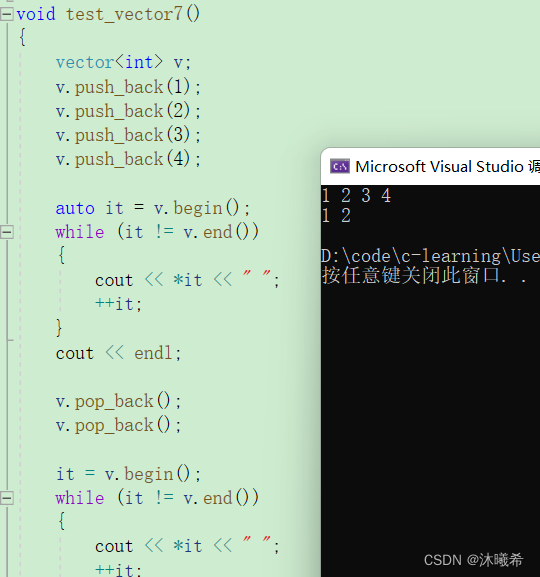• find
find查找函数由算法库函数提供的一个函数模板，使用时需要包含algorithm头文件。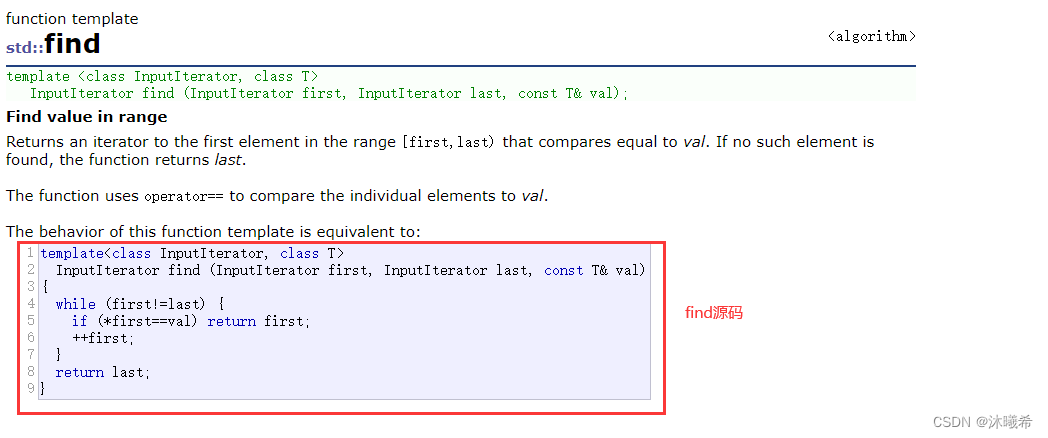``````void test2()
{
vector<int> v;
v.push_back(10);
v.push_back(20);
v.push_back(30);
v.push_back(40);
v.push_back(50);
vector<int>::iterator pos = find(v.begin(), v.end(), 30);
//auto pos = find(v.begin(), v.end(), 30);
if (pos != v.end())
{
cout << "找到了" << endl;
cout << pos - v.begin() << endl;
}
else
{
cout << "没找到" << endl;
}

}
``````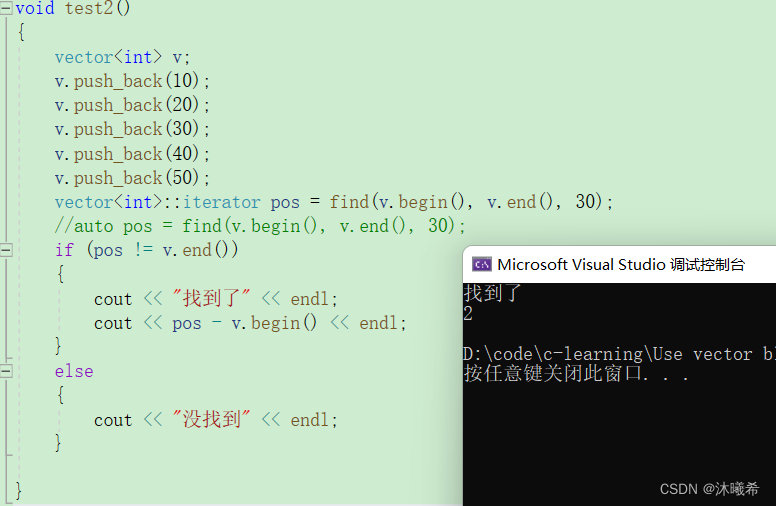AS 打release包

• insert和erase
``````void test_vector8()
{
// 使用列表方式初始化，C++11新语法
vector<int> v{ 1, 2, 3, 4 };
// 在指定位置前插入值为val的元素，比如：3之前插入30,如果没有则不插入
// 1. 先使用find查找3所在位置
// 注意：vector没有提供find方法，如果要查找只能使用STL提供的全局find
auto pos = find(v.begin(), v.end(), 3);
if (pos != v.end())
{
// 2. 在pos位置之前插入30
v.insert(pos, 30);
}
vector<int>::iterator it = v.begin();
while (it != v.end())
{
cout << *it << " ";
++it;
}
cout << endl;
pos = find(v.begin(), v.end(), 3);
// 删除pos位置的数据
v.erase(pos);
it = v.begin();
while (it != v.end()) {
cout << *it << " ";
++it;
}
cout << endl;
}
``````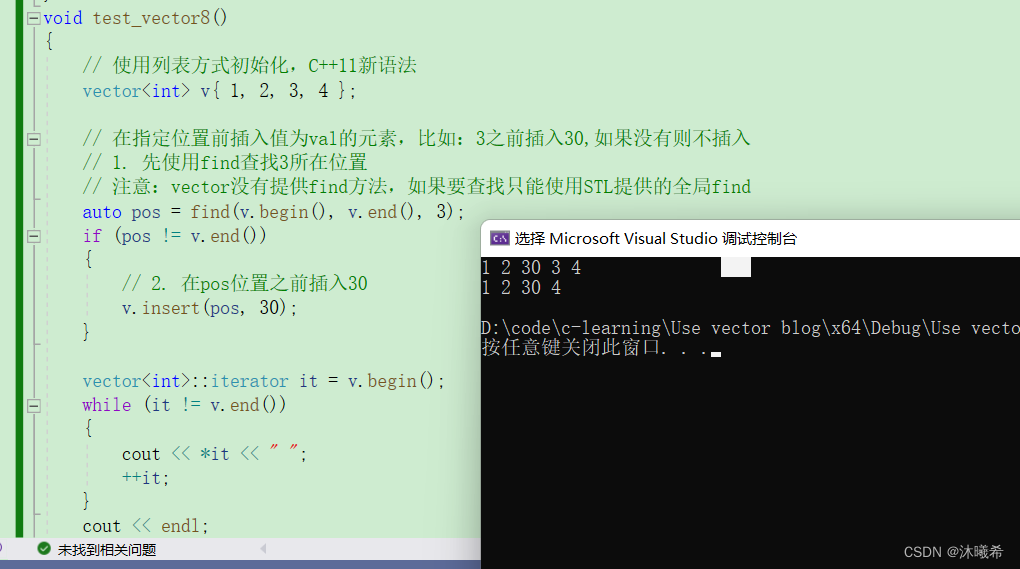AHB_Lite

• swap
交换两个vector类对象的值。
``````void test_vector9()
{
vector<int> v{ 1, 2, 3, 4 };
for (size_t i = 0; i < v.size(); ++i)
cout << v[i] << " ";
cout << endl;
vector<int> swapv;
swapv.swap(v);
cout << "v data:";
for (size_t i = 0; i < v.size(); ++i)
cout << v[i] << " ";
cout << endl;
cout << "swapv data:";
for (auto e : swapv)
{
cout << e << " ";
}
cout << endl;
}
``````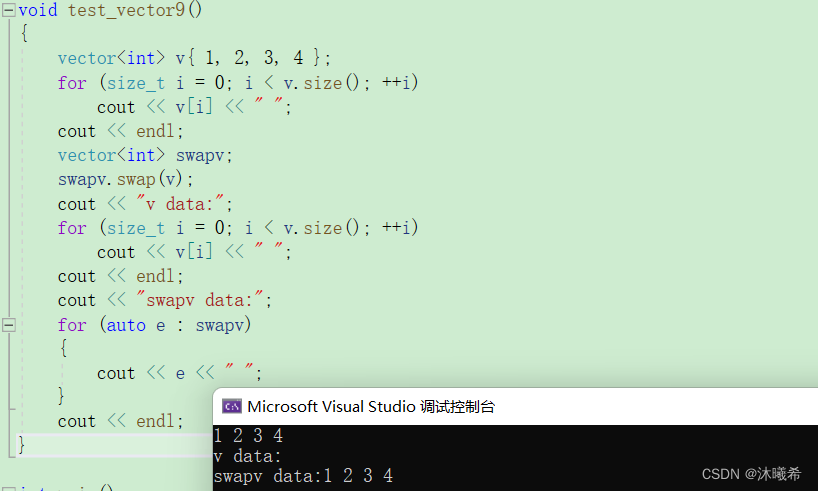# 六、迭代器失效问题

uniapp打包Android

vector可能引起迭代器失效操作：

ESP32-IDF

• 1.会引起其底层空间改变的操作，都有可能是迭代器失效，比如：resize、reserve、insert、assign、push_back等。
``````#include<iostream>
#include<vector>
using namespace std;
int main()
{
vector<int> v{ 1,2,3,4,5,6 };
auto it = v.begin();
// 将有效元素个数增加到100个，多出的位置使用8填充，操作期间底层会扩容
//v.resize(100, 8);
// reserve的作用就是改变扩容大小但不改变有效元素个数，操作期间可能会引起底层容量改变
//v.reserve(100);
// 插入元素期间，可能会引起扩容，而导致原空间被释放
//v.insert(v.begin(), 0);
//v.push_back(8);
// 给vector重新赋值，可能会引起底层容量改变
v.assign(100, 8);
while (it != v.end())
{
cout << *it << " ";
++it;
}
cout << endl;
return 0;
}
``````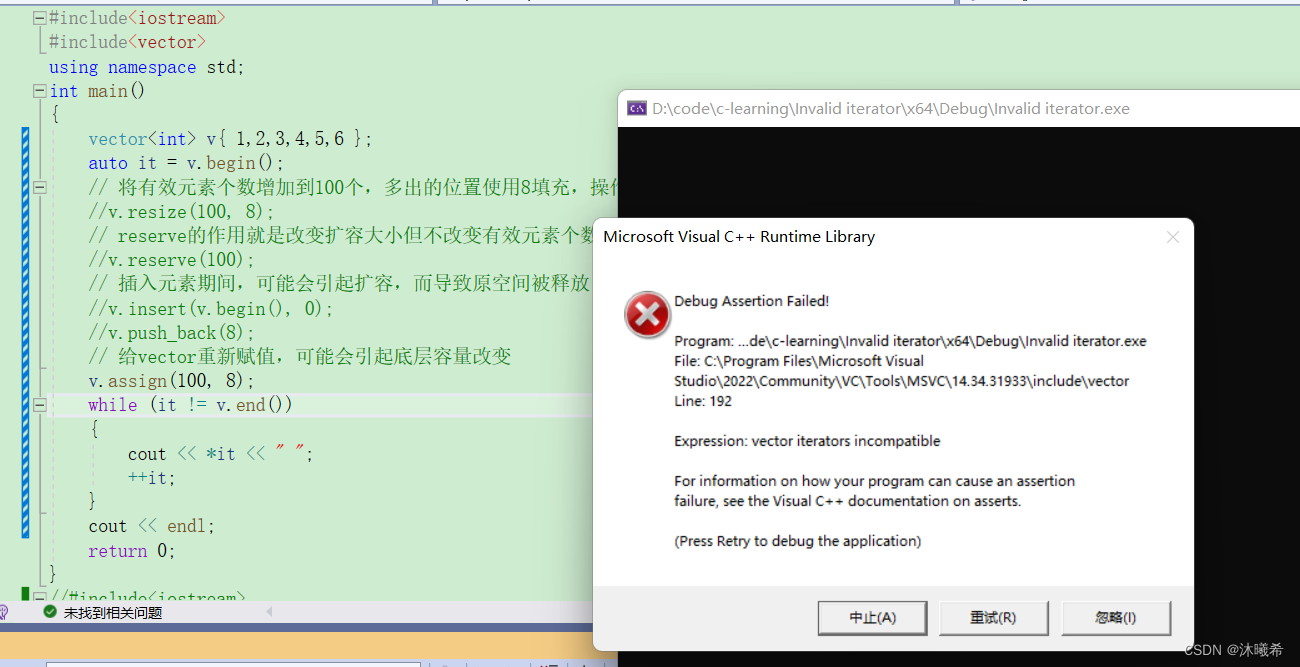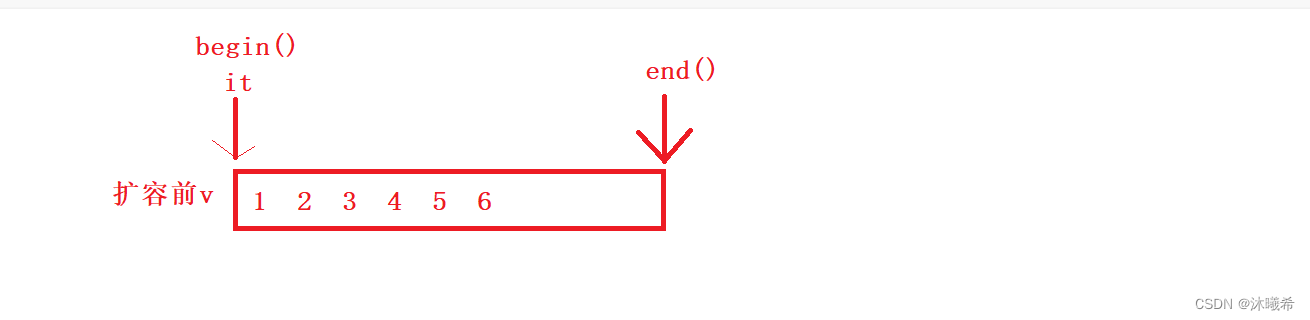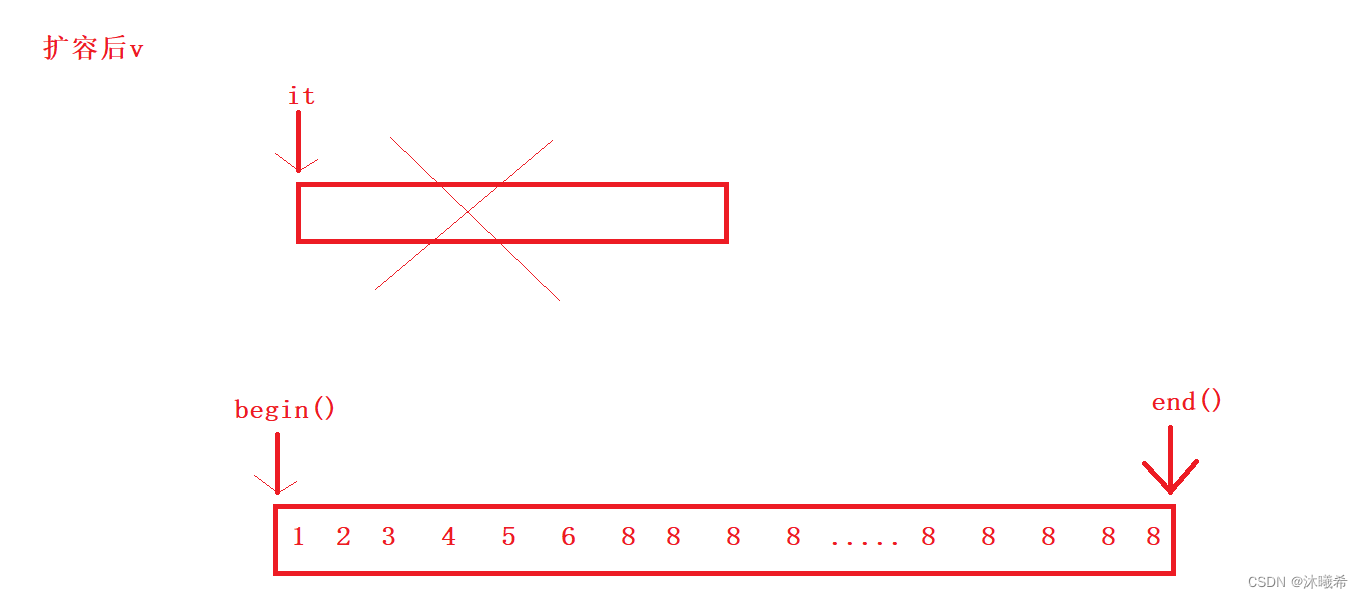springcloud

Iceberg

``````it = v.begin();
while (it != v.end())
{
cout << *it << " ";
++it;
}
cout << endl;
``````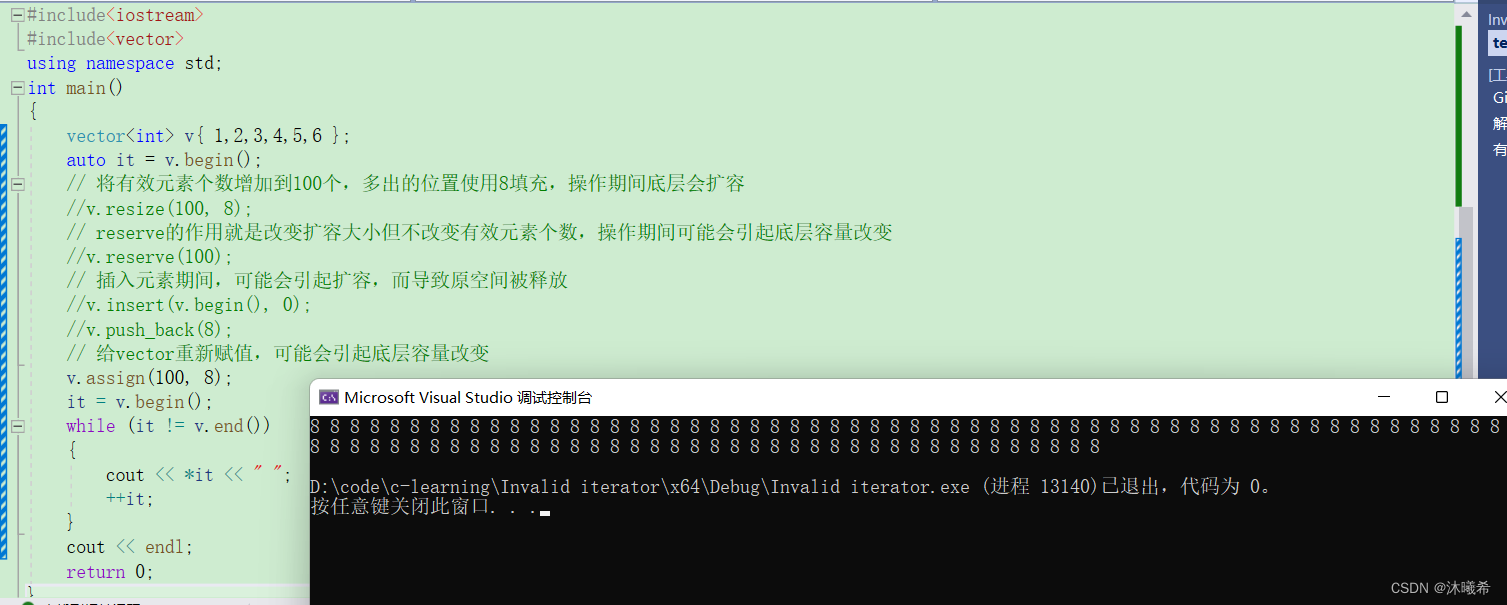mysql 触发器

• 2.指定位置元素的删除操作–erase
``````#include <iostream>
using namespace std;
#include <vector>
int main()
{
int a[] = { 1, 2, 3, 4 };
vector<int> v(a, a + sizeof(a) / sizeof(int));
// 使用find查找3所在位置的iterator
vector<int>::iterator pos = find(v.begin(), v.end(), 3);
// 删除pos位置的数据，导致pos迭代器失效。
v.erase(pos);
cout << *pos << endl; // 此处会导致非法访问
return 0;
}
``````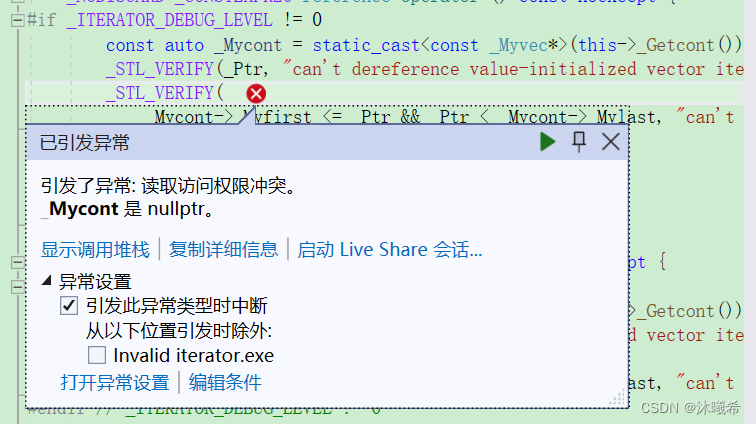erase删除pos位置元素后，pos位置之后的元素会往前搬移，没有导致底层空间的改变，理论上讲迭代器不应该会失效，但是：如果pos刚好是最后一个元素，删完之后pos刚好是end的位置，而end位置是没有元素的，那么pos就失效了。因此删除vector中任意位置上元素时，vs就认为该位置迭代器失效了。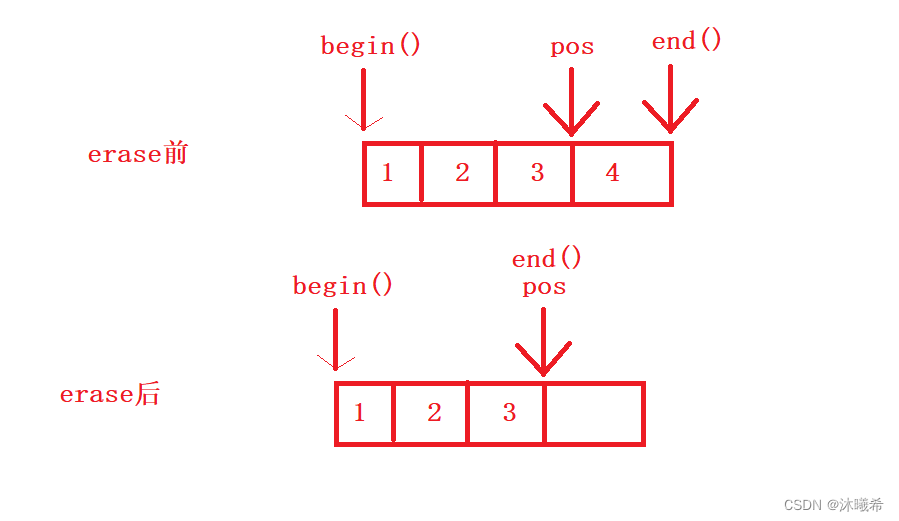• 3.Linux下，g++编译器对迭代器失效的检测并不是非常严格，处理也没有vs下极端
``````#include<iostream>
#include<vector>
using namespace std;
// 1. 扩容之后，迭代器已经失效了，程序虽然可以运行，但是运行结果已经不对了
int main()
{
vector<int> v{1,2,3,4,5};
for(size_t i = 0; i < v.size(); ++i)
cout << v[i] << " ";
cout << endl;
auto it = v.begin();
cout << "扩容之前，vector的容量为: " << v.capacity() << endl;
// 通过reserve将底层空间设置为100，目的是为了让vector的迭代器失效
v.reserve(100);
cout << "扩容之后，vector的容量为: " << v.capacity() << endl;
// 经过上述reserve之后，it迭代器肯定会失效，在vs下程序就直接崩溃了，但是linux下不会
// 虽然可能运行，但是输出的结果是不对的
while(it != v.end())
{
cout << *it << " ";
++it;
}
cout << endl;
return 0;
}
``````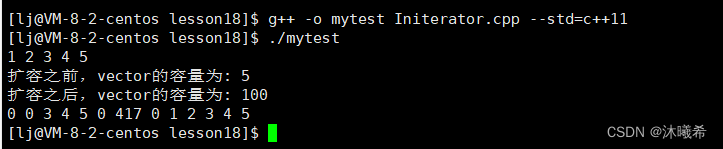``````// 2. erase删除任意位置代码后，linux下迭代器并没有失效
// 因为空间还是原来的空间，后序元素往前搬移了，it的位置还是有效的
#include<iostream>
#include <vector>
#include <algorithm>
using namespace std;
int main()
{
vector<int> v{1,2,3,4,5};
vector<int>::iterator it = find(v.begin(), v.end(), 3);
v.erase(it);
return 0;
}
``````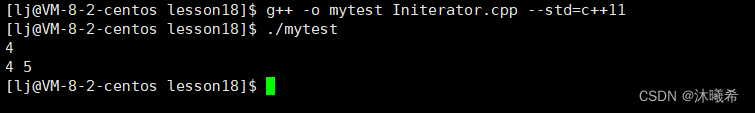``````// 3: erase删除的迭代器如果是最后一个元素，删除之后it已经超过end
// 此时迭代器是无效的，++it导致程序崩溃
#include<iostream>
#include<vector>
using namespace std;
int main()
{
vector<int> v{1,2,3,4,5};
// vector<int> v{1,2,3,4,5,6};
auto it = v.begin();
while(it != v.end())
{
if(*it % 2 == 0)
v.erase(it);
++it;
}
for(auto e : v)
cout << e << " ";
cout << endl;
return 0;
}
``````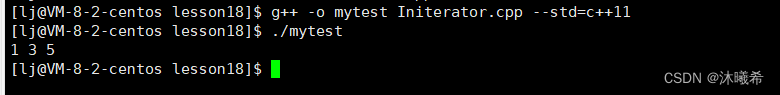• 4.与vector类似，string在插入+扩容操作+erase之后，迭代器也会失效
``````#include<iostream>
#include <string>
using namespace std;
void TestString()
{
string s("hello");
auto it = s.begin();
// 放开之后代码会崩溃，因为resize到20会string会进行扩容
// 扩容之后，it指向之前旧空间已经被释放了，该迭代器就失效了
// 后序打印时，再访问it指向的空间程序就会崩溃
//s.resize(20, '!');
while (it != s.end())
{
cout << *it;
++it;
}
cout << endl;
it = s.begin();
while (it != s.end())
{
it = s.erase(it);
// 按照下面方式写，运行时程序会崩溃，因为erase(it)之后
// it位置的迭代器就失效了
// s.erase(it);
++it;
}
}
int main()
{
TestString();
return 0;
}
``````